Question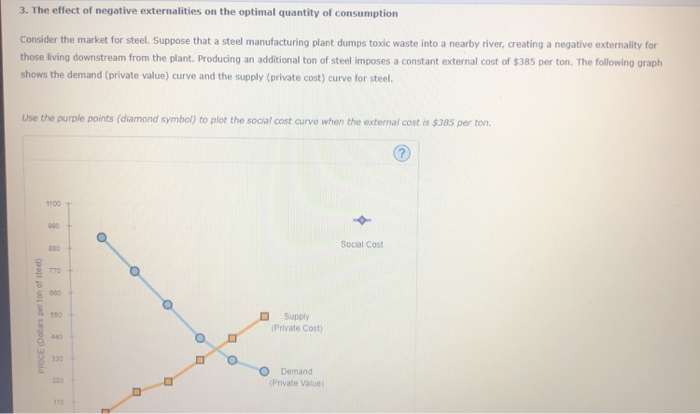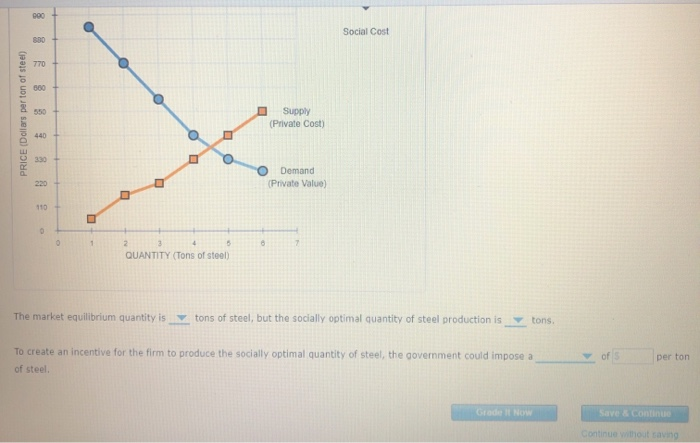It is provided that the each additional ton of steel production imposes a constant external cost of \$385 per ton.

So,

This will shift the private cost curve upward by \$385 at each ton of steel produced.

Following is the required figure -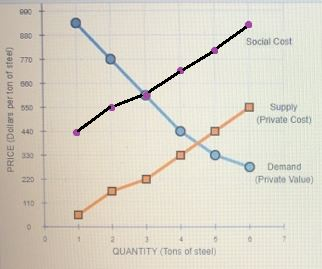The supply (private cost) and demand (private value) are intersecting each other corresponding to output of 4.5 tons of steel.

On the other hand, the social cost curve and demand (private value) curve are intersecting each other corresponding to output of 3 tons of steel.

So,

The market equilibrium quantity is 4.5 tons of steel, but the socially optimal quantity of steel production is 3 tons.

To create an incentive for the firm to produce the socially optimal quantity of steel, the government could impose a tax of \$385 per ton of steel.

#### Earn Coins

Coins can be redeemed for fabulous gifts.

Similar Homework Help Questions
• ### 3. The effect of negative externalities on the optimal quantity of consumption Consider the market for...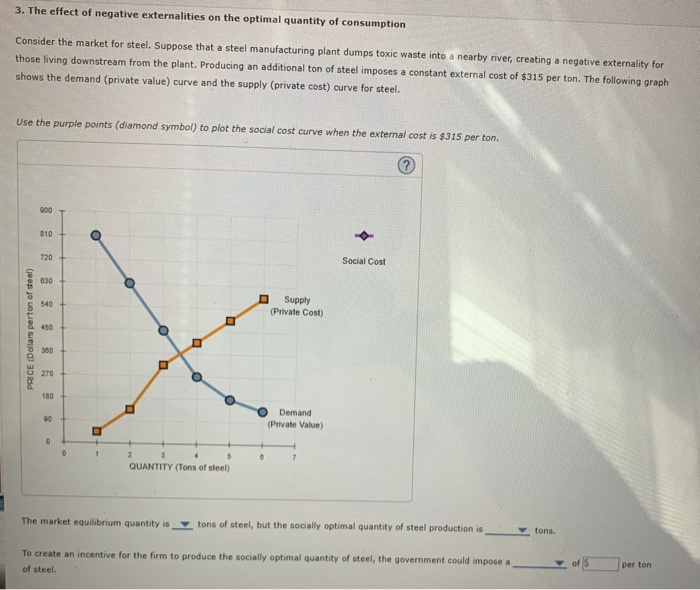3. The effect of negative externalities on the optimal quantity of consumption Consider the market for steel. Suppose that a steel manufacturing plant dumps toxic waste into a nearby river, creating a negative externality for those living downstream from the plant. Producing an additional ton of steel imposes a constant external cost of \$315 per ton. The following graph shows the demand (private value) curve and the supply (private cost) curve for steel. Use the purple points (diamond symbol) to...

• ### 3. The effect of negative externalities on the optimal quantity of consumption Consider the market for...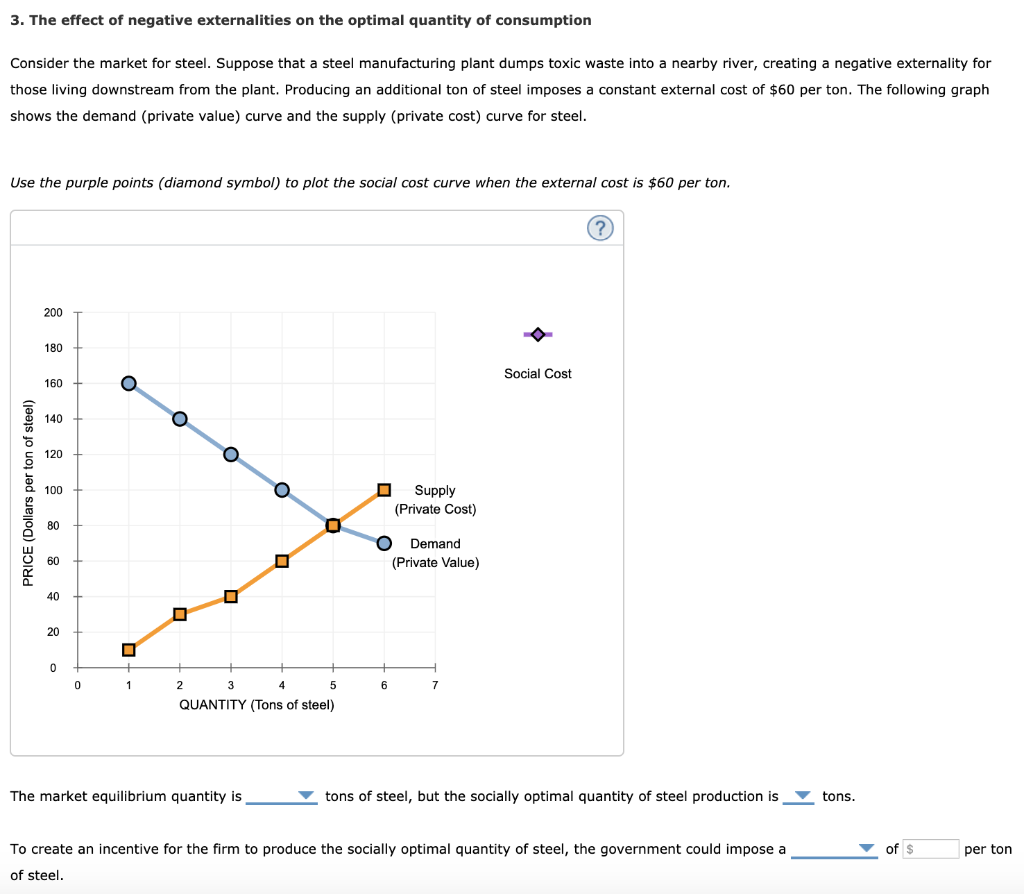3. The effect of negative externalities on the optimal quantity of consumption Consider the market for steel. Suppose that a steel manufacturing plant dumps toxic waste into a nearby river, creating a negative externality for those living downstream from the plant. Producing an additional ton of steel imposes a constant external cost of \$60 per ton. The following graph shows the demand (private value) curve and the supply (private cost) curve for steel. Use the purple points (diamond symbol) to...

• ### 3. The effect of negative externalities on the optimal quantity of consumption Consider the market for...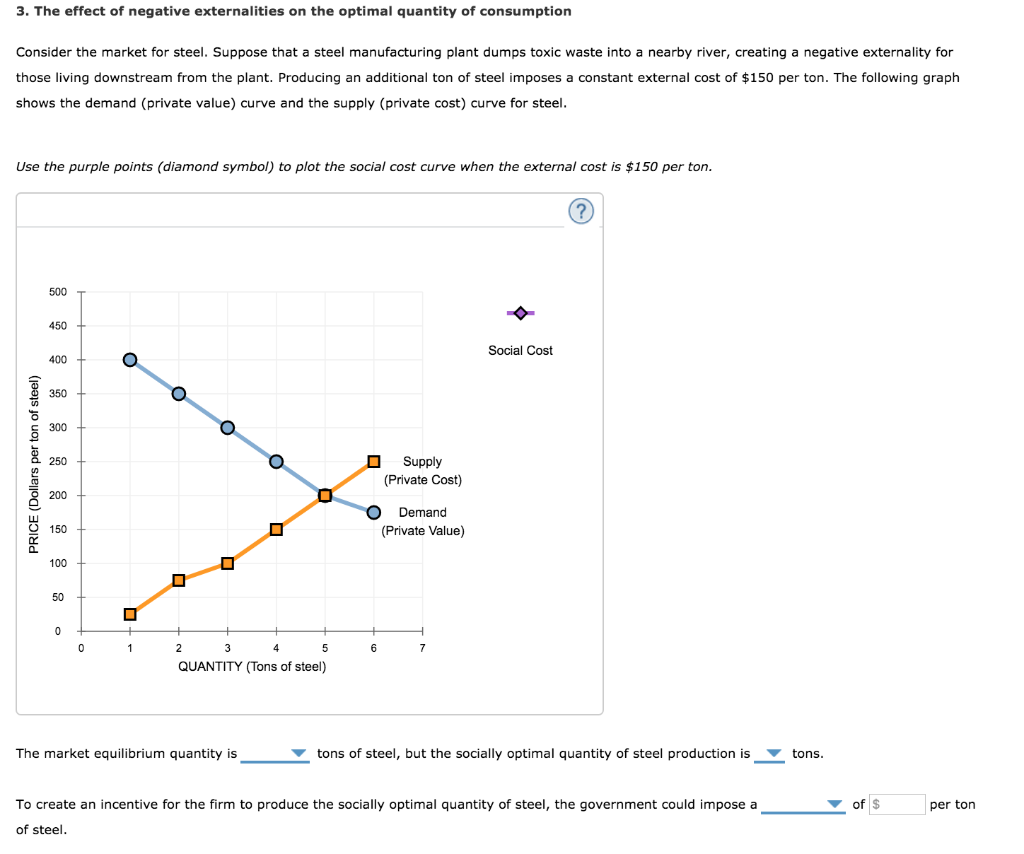3. The effect of negative externalities on the optimal quantity of consumption Consider the market for steel. Suppose that a steel manufacturing plant dumps toxic waste into a nearby river, creating a negative externality for those living downstream from the plant. Producing an additional ton of steel imposes a constant external cost of \$150 per ton. The following graph shows the demand (private value) curve and the supply (private cost) curve for steel. Use the purple points (diamond symbol) to...

• ### 3. The effect of negative externalities on the optimal quantity of consumption Consider the market for...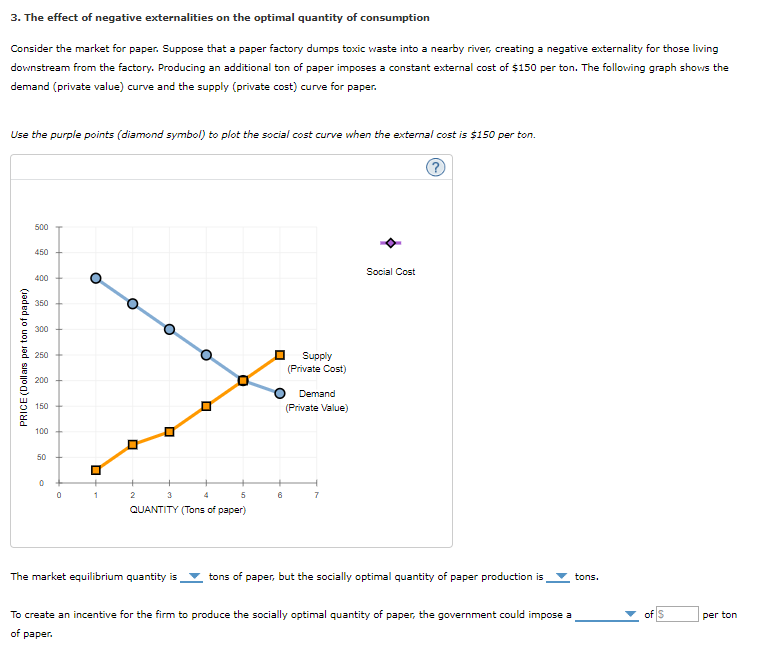3. The effect of negative externalities on the optimal quantity of consumption Consider the market for paper. Suppose that a paper factory dumps toxic waste into a nearby river, creating a negative externality for those living downstream from the factory. Producing an additional ton of paper imposes a constant external cost of \$150 per ton. The following graph shows the demand (private value) curve and the supply (private cost) curve for paper. Use the purple points (diamond symbol) to plot...

• ### 3. The effect of negative externalities on the optimal quantity of consumption Consider the market for...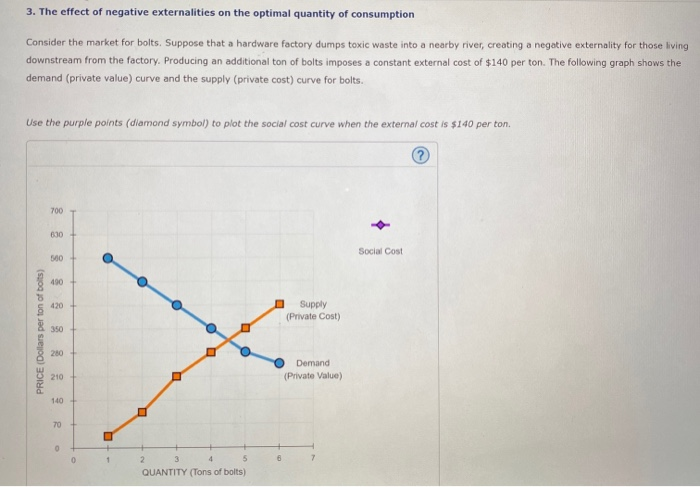3. The effect of negative externalities on the optimal quantity of consumption Consider the market for bolts. Suppose that a hardware factory dumps toxic waste into a nearby river, creating a negative externality for those living downstream from the factory. Producing an additional ton of bolts imposes a constant external cost of \$140 per ton. The following graph shows the demand (private value) curve and the supply (private cost) curve for bolts. Use the purple points (diamond symbol) to plot...

• ### Homework (Ch 10) 3. The effect of negative externalities on the optimal quantity of consumption Consider the market...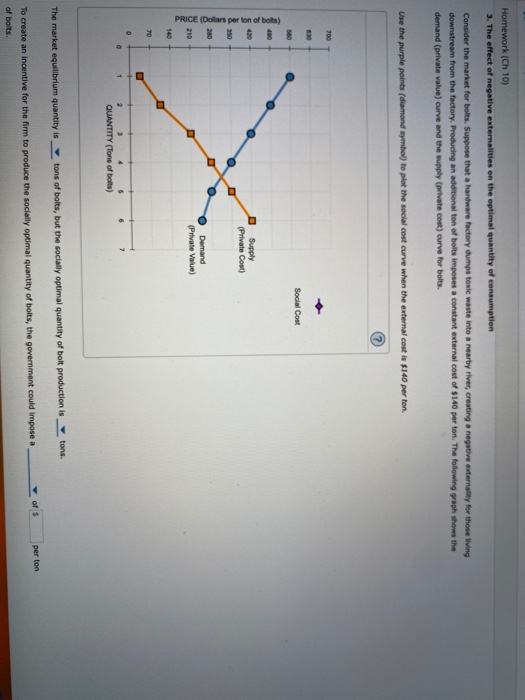Homework (Ch 10) 3. The effect of negative externalities on the optimal quantity of consumption Consider the market for bolts. Suppose that a hardware factory dumps toxic waste into a nearby river, creating a negative externality for those living downstream from the factory Producing an additional ton of bolts imposes a constant external cost of \$140 per ton. The following graph shows the demand (private value) curve and the supply (private cost) curve for bolts. Use the purple points (diamond...

• ### 3. The effect of negative externalities on the optimal quantity of consumption Consider the market for...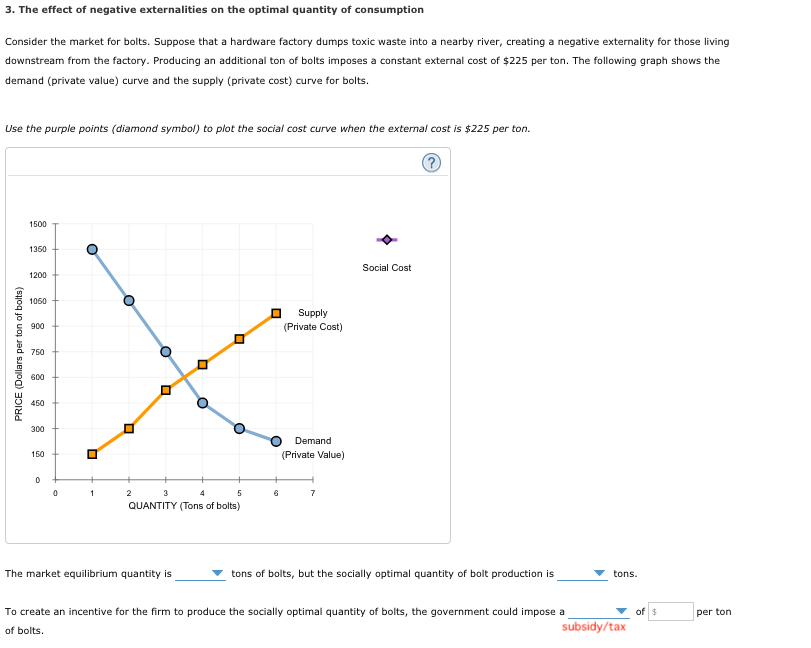3. The effect of negative externalities on the optimal quantity of consumption Consider the market for bolts. Suppose that a hardware factory dumps toxic waste into a nearby river, creating a negative externality for those living downstream from the factory. Producing an additional ton of bolts imposes a constant external cost of \$225 per ton. The following graph shows the demand (private value) curve and the supply (private cost) curve for bolts Use the purple points (diamond symbol) to plot...

• ### The effect of negative externalities on the optimal quantity of consumption Consider the market for bolts. Suppose that...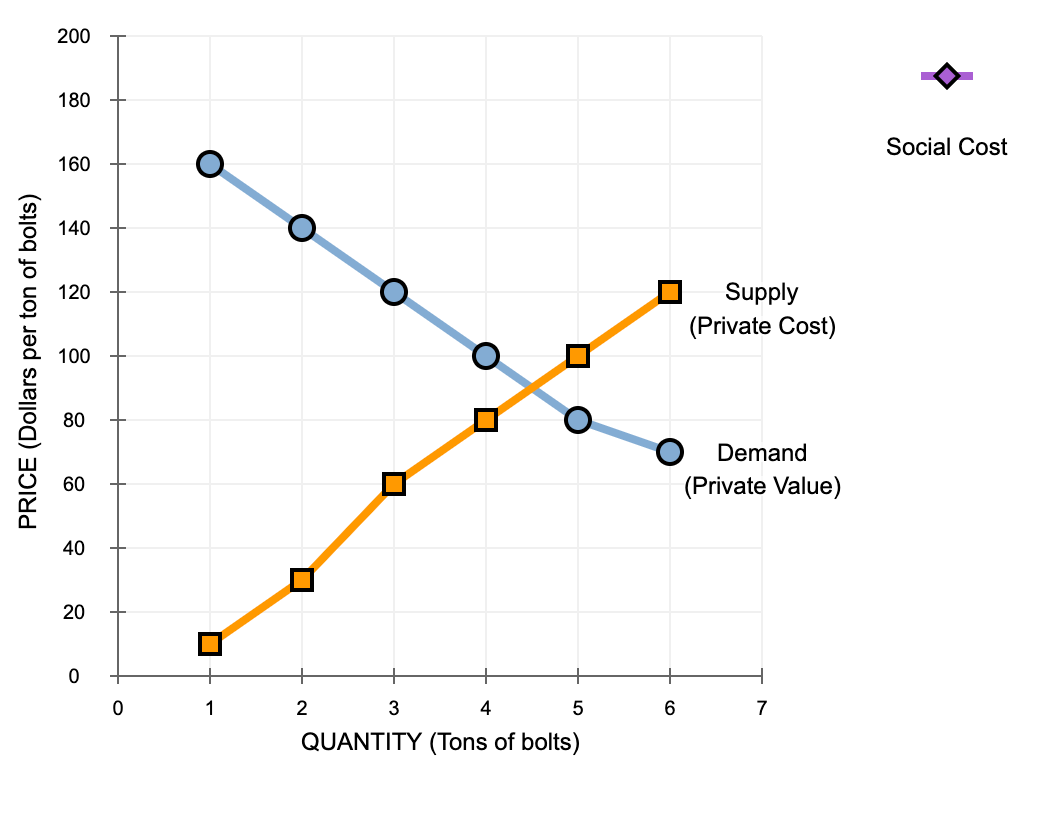The effect of negative externalities on the optimal quantity of consumption Consider the market for bolts. Suppose that a hardware factory dumps toxic waste into a nearby river, creating a negative externality for those living downstream from the factory. Producing an additional ton of bolts imposes a constant external cost of \$40 per ton. The following graph shows the demand (private value) curve and the supply (private cost) curve for bolts. 1. plot the social cost curve when the external...

• ### 3. The effect of negative externalities on the optimal quantityof consumption Consider the market for bolts....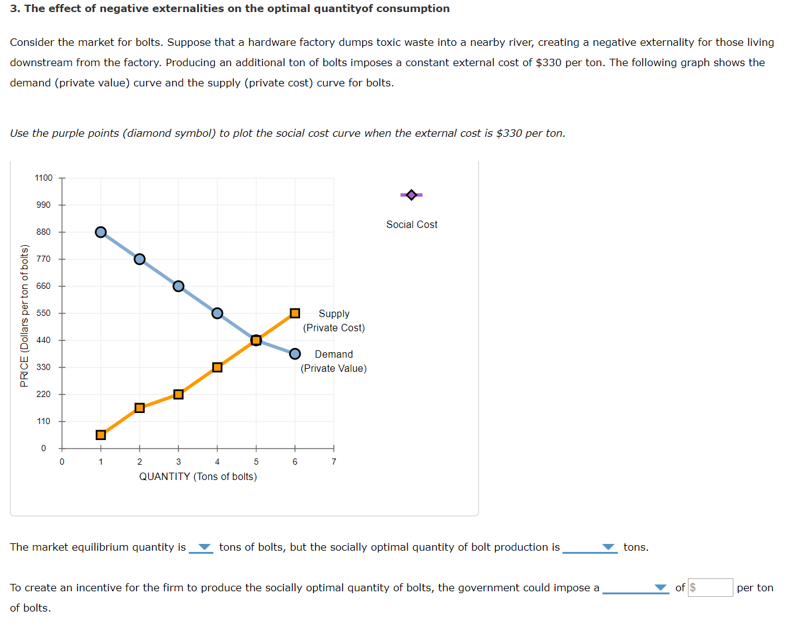3. The effect of negative externalities on the optimal quantityof consumption Consider the market for bolts. Suppose that a hardware factory dumps toxic waste into a nearby river, creating a negative externality for those living downstream from the factory. Producing an additional ton of bolts imposes a constant external cost of \$330 per ton. The following graph shows the demand (private value) curve and the supply (private cost) curve for bolts. Use the purple points (diamond symbol) to plot the...

• ### 3. The effect of negative externalities on the optimal quantityof consumption Consider the market for paper....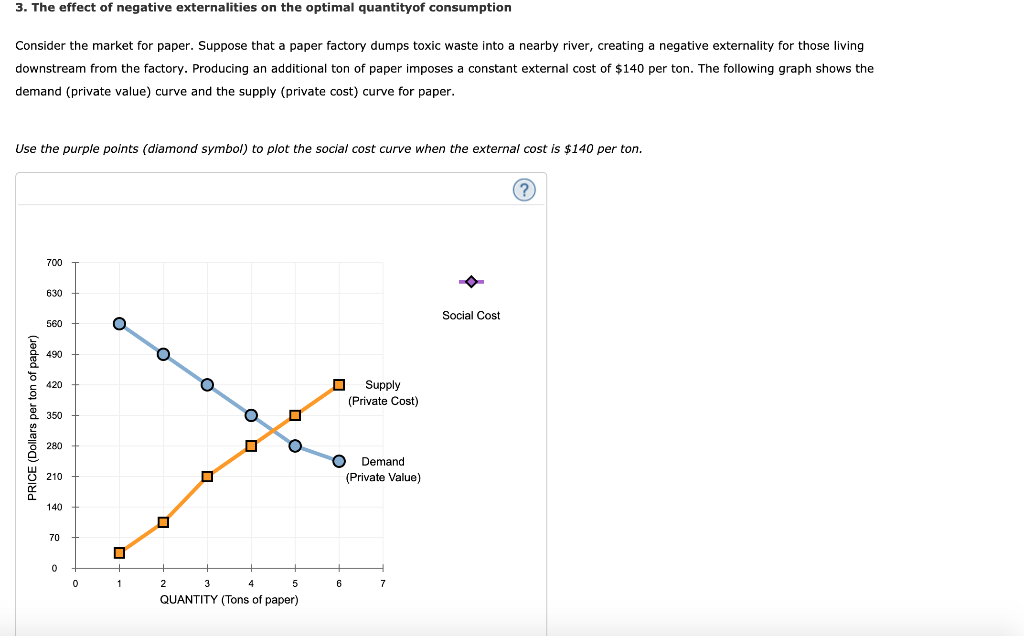3. The effect of negative externalities on the optimal quantityof consumption Consider the market for paper. Suppose that a paper factory dumps toxic waste into a nearby river, creating a negative externality for those living downstream from the factory. Producing an additional ton of paper imposes a constant external cost of \$140 per ton. The following graph shows the demand (private value) curve and the supply (private cost) curve for paper. Use the purple points (diamond symbol) to plot the...